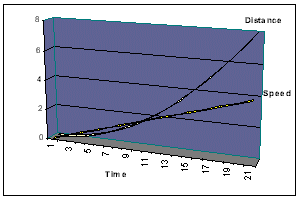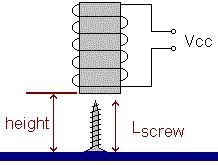# Coil Size

How do you choose the size of the coil? How can you tell if you've made it strong enough?

How can you measure the strength of a given coil? How can you predict the acceleration of a screw or nail within the coil? How can you tell if a dog's mouth is really cleaner than a human's?

Ah well, computing technology probably has an answer somewhere. There's probably some program that can calculate all this for you. This may even be one of the best studied areas of electromagnetics, since it's at the heart of common solenoid design. Engineers have been designing solenoids for, what, 100 years now? I suspect the design parameters are so old and simple that you can't even find them in reference books any longer. However, the interesting stuff happens in the air gaps where fringing occurs, and it's mathematically hard to analyze fringing. I've forgotten how to do surface integrals of anything, much less a screw or nail.

So... I built some coils. Here's how I took measurements and calculated the attractive strength for a given applied voltage. From that, spreadsheet #1 computed the force of acceleration at each incremental position within the coil. Given all this, spreadsheet #2 computed the speed and timing at each millimeter position as a virtual projectile streaks through the coil.

## Setup to Measure Coil Strength

You need to know the attractive force at each position as the projectile enters the coil. The force will be near zero when the steel projectile is outside the coil. The force should increase proportionally to the amount of steel inside the coil. It tends to pull the steel to the midpoint of the coil length; therefore we'll call the force positive as the steel is introduced to the magnetic field, and negative after the steel goes past the midpoint. Looking ahead, in a real coil gun we need to turn off the coil before the projectile reaches the midpoint.

So here's some ideas. I've come up with one way to measure these forces with a minimum of laboratory equipment. All you need is a ruler and an adjustable dc power supply. Suppose the projectile is a flat-head screw (so it can stand up by itself). Stand the screw with the tip pointing up, and position the coil at certain measured distances above it. At each position, slowly increase the applied voltage until the screw is lifted off the table.This method yields acceleration directly. You don't need to know the mass of the projectile, or the force in grams / ounces / pounds / tons / newtons. You don't have to weigh it because "one gee" force is our calibration standard. How convenient that your friendly local gravity field provides a free calibrator for you!

You recall that f = ma (force equals mass times acceleration). Instead of measuring tricky little quantities of force and mass in tiny amounts, we'll get the acceleration figure directly. This is what we really want, anyway, to fill into the basic equations of motion:

velocity as function of time: v = at

distance as function of time: d = at2/2.

## Measuring Coil Strength

My method goes like this. First, measure the length of the screw. Then follow these steps:

1.Position the coil above the screw.
2. Measure the height from the table to the coil. (We want the depth of the screw into the coil, but it's hard to measure directly because we can't easily reach inside the coil.) I strapped a micrometer firmly onto the side of the coil to measure the height above the table.